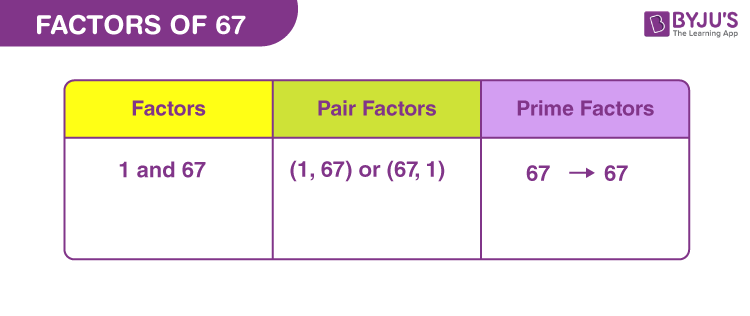# Factors of 67

Factors of 67 can divide the original number, evenly. Factors are the natural numbers which are also called divisors of the original number. Since 67 is a prime number, therefore, it is divisible by 1 and the number itself.

67 is an odd number because it cannot be divided by 2. 67 does not come in any table apart from table of 1 and table of 67. Also, 67 is a special number, which is the sum of five consecutive prime numbers, such that:

7+11+13+17+19 = 67

Where 7, 11, 13, 17 and 19 are the prime numbers.## How to Find the Factors of 67?

To find the factors, divide the number by the natural numbers. Since, the only possibility of divisibility of 67 here is two, therefore:

 67 ÷ 1 = 67 [Remainder = 0] 67 ÷ 67 = 1 [Remainder = 0]

Therefore, there are only two factors of 67. They are 1 and 67.

Let us see if 67 is divisible by any other number.

67 ÷ 2 = 33.5

67 ÷ 5 = 13.4

67 ÷ 11 = 6.09090909091

67 ÷ 25 = 2.68

From the above examples, we can see that we are not able to determine any other factors of 67. Hence, 67 is only divisible by 1 and 67.

## Pair Factors of 67

Pair factors result in the original number, when multiplied in pairs. Since we have only two factors of 67 here, thus only two pairs of factors are possible.

• 1 × 67 = 67
• 67 × 1 = 67

Therefore, the pair factors of 67 are (1, 67) or (67, 1).

We can also consider the negative pair of numbers to get the result.

• -1 × -67 = 67
• -67 × -1 = 67

Therefore, the negative pair factors are (-1, -67) or (-67, -1).

## Prime Factorisation of 67

The prime factorisation of 67 is not possible because 67 itself is a prime number. Therefore, it is divisible by only one prime number, i.e. itself.

67 ÷ 67 = 1

 Prime factorisation of 67 = 67 Exponential form = 671

## Solved Examples

Q.1: What is the sum of all the factors of 67?

Solution: The factors of 67 are 1 and 67.

Sum = 1 + 67 = 68

Therefore, the required sum is 68.

Q.2: What are the GCF of 67 and 71?

Solution: We have to list the factors of 67 and 71.

67 → 1, 67

71 → 1, 71

Therefore, the GCF of 67 and 71 is 1.

Q.3: What are the common factors of 67 and 201?

Solution: The factors of respective numbers are:

67 → 1, 67

201 → 1, 3, 67, 201

Therefore, the common factors of 67 and 201 are 1 and 67.

### Practice Questions

1. What are the divisors of 67?
2. What is the average of factors of 67?
3. What is the GCF of 67 and 68?
4. How many factors does 67 have?

## Frequently Asked Questions on Factors of 67

### What are the factors of 67?

The factors of 67 are 1 and 67.

### Is 67 a prime number?

Yes, 67 is a prime number because it has only two factors.

### What are the multiples of 67?

The multiples of 67 are 67, 134, 201, 268, 335, 402, 469, 536, 603 and 670.

### Is 67 divisible by any other number?

67 is not divisible by any other number apart from 1 and 67

### Is 67 a perfect square?

67 is not a perfect square, since the square of no natural number will result in 67.